# Tracking Emerges by Colorizing Videos

## WHY?

Segmenting objects in videos is difficult without manual labels.

## WHAT?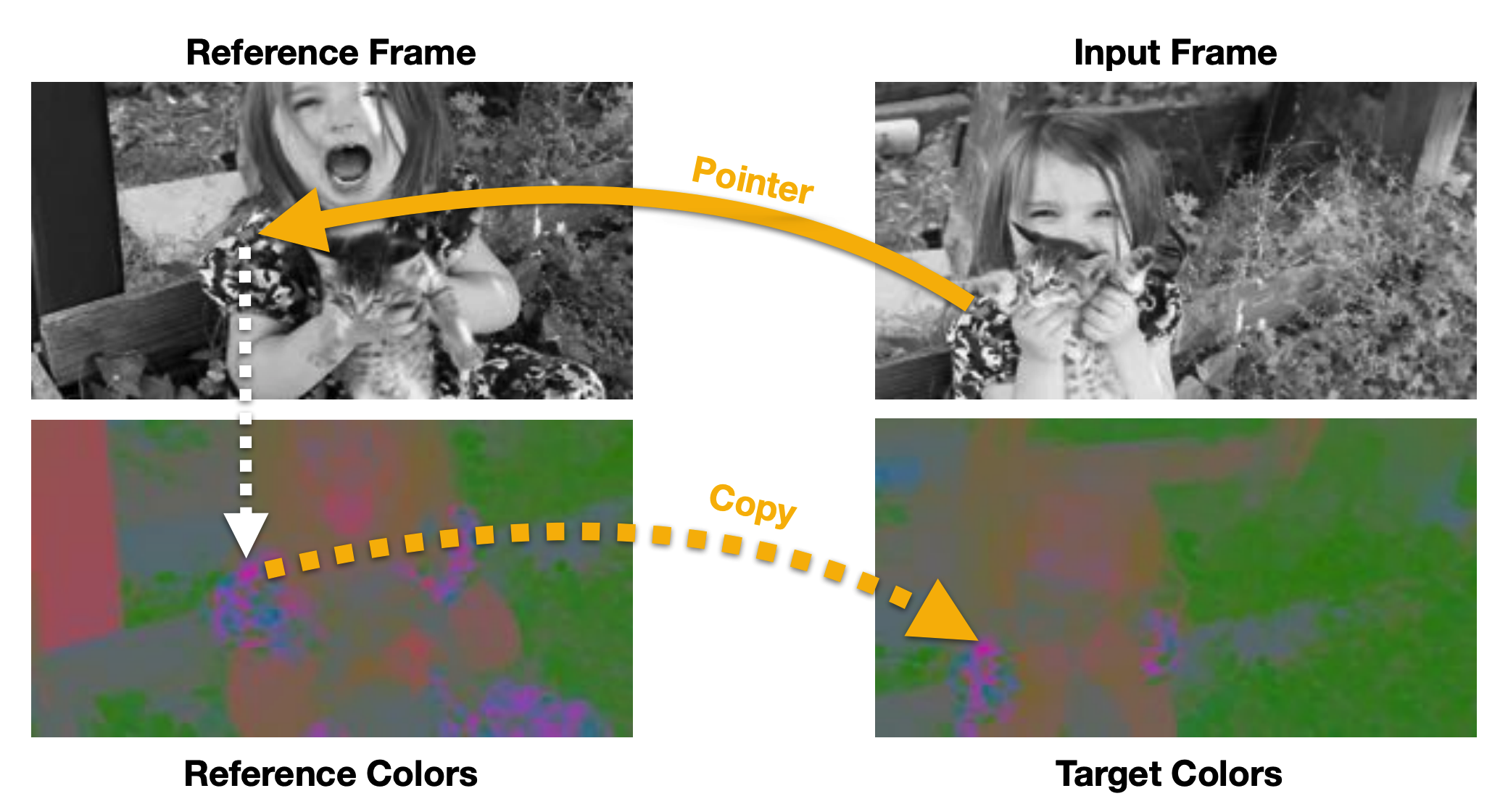This paper suggests to learn segmenting the objects in video sequence with model trained with self-supervised colorizing task.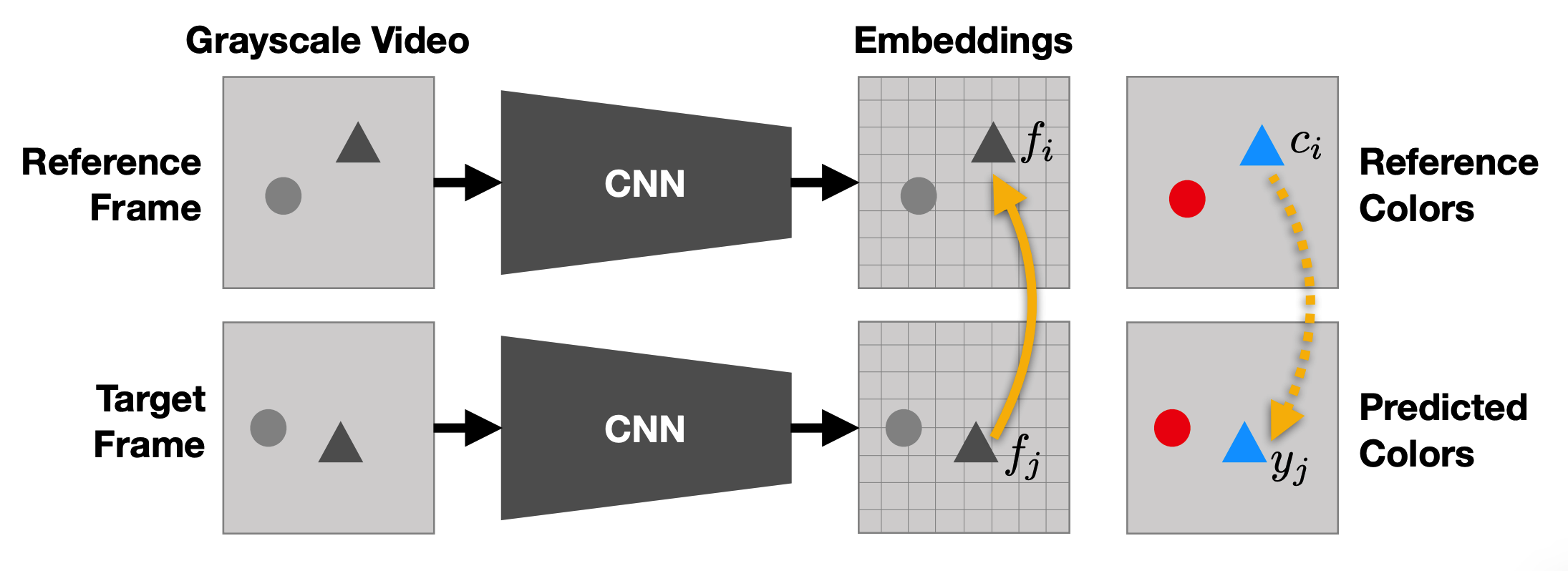Given reference frames and a grayscale input frame, the model tries to learn the color of the grayscale input frame from reference frames. To do this, model gets embeddings of each of pixels in frames with convolutional neural network. The model estimates the color of a pixel in input frame with the sum of colors of reference frames weighted by similarity of embedding. Colors are quantized with k-means to formulate the estimation as categorization.

y_j = \sum_i A_{ij}c_i\\
A_{ij} = \frac{exp(f_i^T f_j)}{\sum_k exp(f_k^T f_j)}\\
min_{\theta}\sum_j\mathcal{L}(y_j, c_j)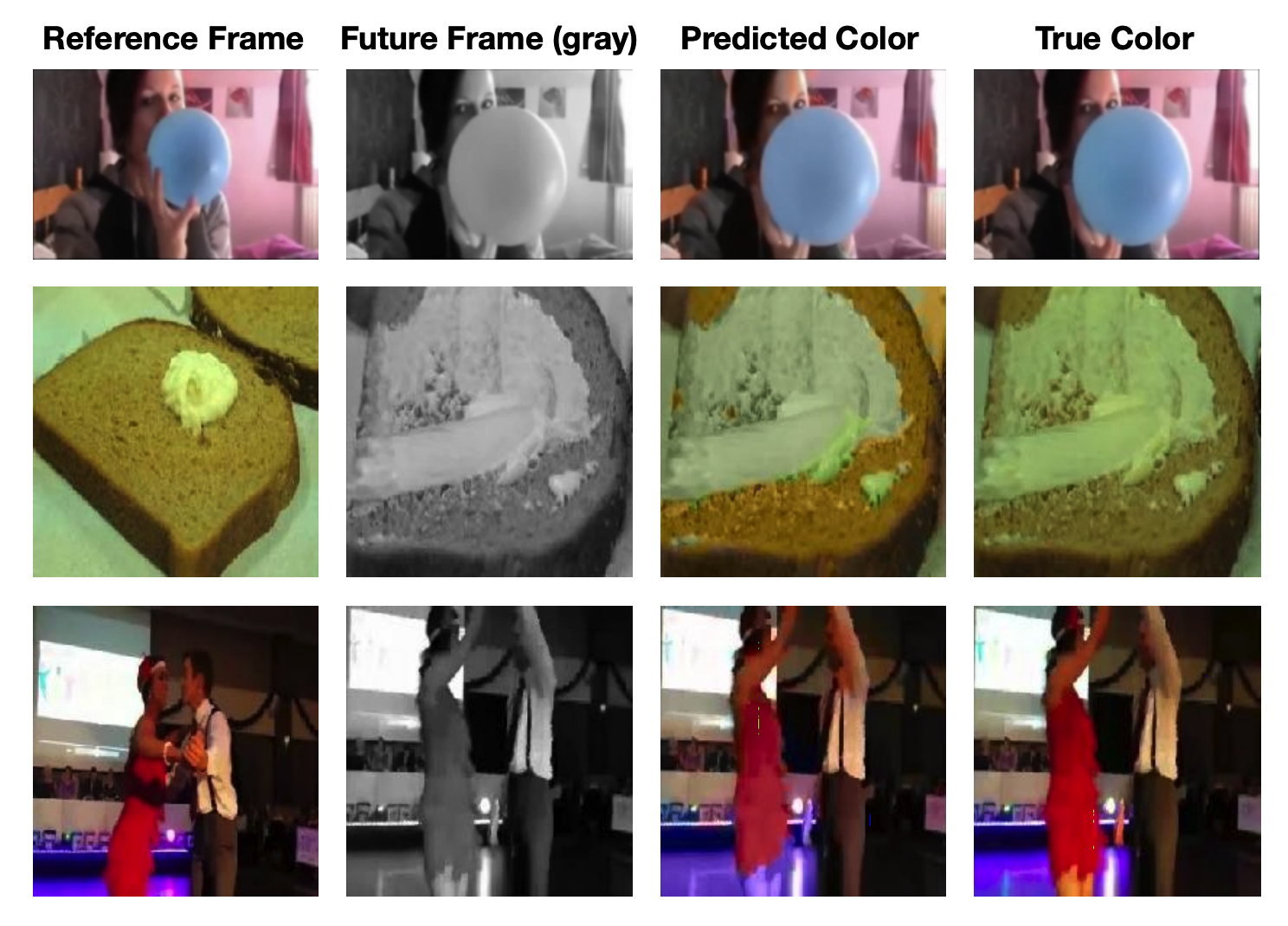With the learned colorizing model, segments or keypoints can be tracked with the labels on the reference frame which is formulated as categorization.

## So?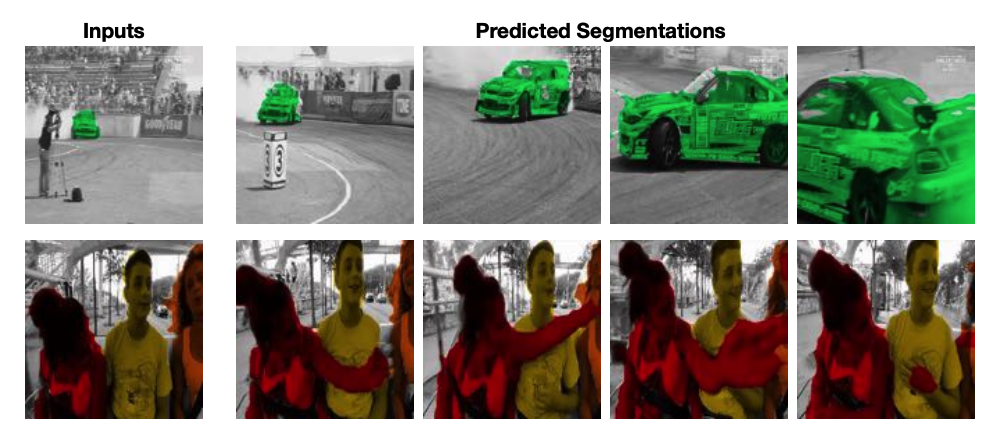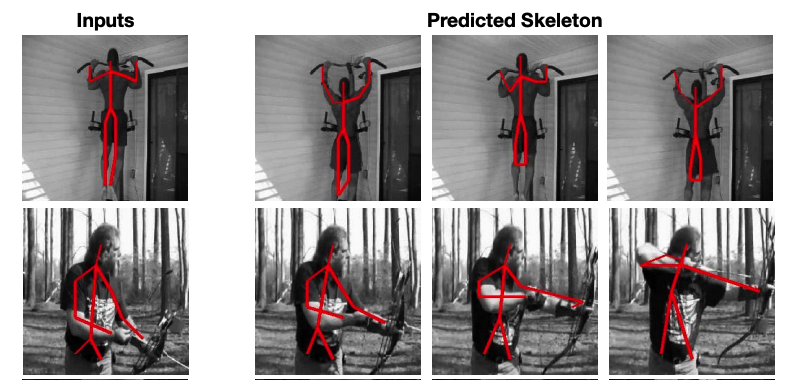This model showed good performance on the segmentation and pose tracking.

Powered by aiden